Next: Bernoulli's equation Up: Steady flows of incompressible Previous: Steady flows of incompressible

Ideal fluids and Euler's equation

To obtain the equation of motion for a fluid we appeal to Newton's Second Law--the mass of a fluid element times its acceleration is equal to the net force acting on that fluid element. If we take an element of unit volume, then we have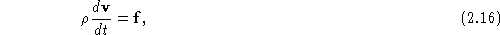where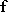is the force per unit volume on a fluid element. This force may have several contributions. The first is the ``internal'' force which is due to viscous dissipation, which we will ignore for right now. The second set are ``body forces'' which act throughout the volume of the fluid, such as the gravitational force. The third force is due to pressure gradients within the fluid. To see how this works, consider a cube of fluid, with dimensions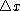,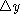, and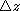, as shown in Fig. 2.6.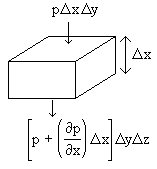Figure 2.6: Derivation of the force on a fluid element due to pressure gradients.

The force on the top face at position x is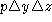, while the force on the bottom face is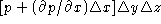; subtracting, we see that the net force in the x-direction is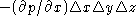, so the pressure per unit volume is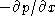. Repeating for the y and z directions, we find the net force per unit volume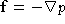. Therefore, if we ignore viscosity and gravity for the moment, we have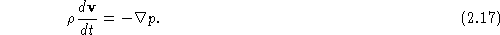Now comes the tricky part. What is the acceleration of the fluid? We want the acceleration of a particular element of the fluid; the coordinates of this fluid element change in time as the fluid flows. In a time interval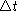, the x-coordinate changes by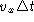, the y-coordinate by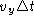, and the z-coordinate by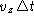. The velocity then becomes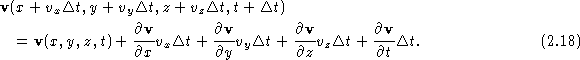To calculate the acceleration, we need to find the rate of change of the velocity:We see that the acceleration is not simply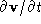. The reason for this is that even if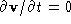, so that the velocity at a given point is not changing, that doesn't mean that a fluid element is not accelerating. A good example is circular flow in a bucket. If the flow is steady, then at a point in the bucket, even though a fluid element in the bucket is experiencing a centripetal acceleration. The term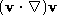is nonlinear, and is the source of all of the difficulties in fluid mechanics. Pulling together all of the pieces, we have for our equation of motionThis is known as Euler's equation . This equation and the equation of continuity are the governing equations of nonviscous fluid flow.

For an incompressible fluid, the Euler equation can be written in a somewhat different form which is often useful for applications. We use the following identity from vector calculus: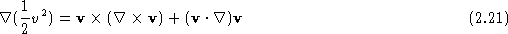[see the PQRG, p. 161, next to last equation]. Next we use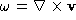, and the fact that the fluid is incompressible so thatis constant, to rewrite Eq. (2.20) as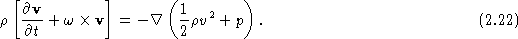This is still a complicated equation, but it has interesting consequences. If we apply Ñ× to both sides of this equation, and remenber that the curl of a gradient is zero, we obtain an equation that tells us how the vorticity moves:

w/t + Ñ×(w×v) = 0.

In particular, this equation shows that w remains zero at all times if it is zero initially. It also it allows us to check if some w can stay fixed in time and produce a steady flow.Next: Bernoulli's equation Up: Steady flows of incompressible Previous: Steady flows of incompressible

Notes by A. Dorsey, revised by V. Celli, U. of Virginia
Wed Sep 10 01:02:02 EDT 1997